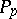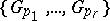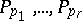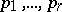Letbe a finite group anda subset of the prime numbers that divide the orderof. A Sylow-basisis a collection of Sylow-subgroupsof(cf. Sylow subgroup), one for each primein, such that: Ifare in, then the order of every element in(the subgroup generated by) is a product of non-negative powers of. Ifis the set of all primes dividing, one speaks of a complete Sylow basis. Two Sylow bases are conjugate if there is a single element ofthat by conjugation transforms all the groups of the first into those of the second. Hall's second theorem, [a2], says that every finite solvable group has a complete Sylow basis, and that all these bases are conjugate. Conversely, if a finite group has a complete Sylow basis, then it is solvable (cf. also Solvable group).